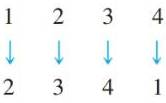Chapter 9.2, Problem 47ES### Discrete Mathematics With Applicat...

5th Edition
EPP + 1 other
ISBN: 9781337694193

#### Solutions

Chapter
Section### Discrete Mathematics With Applicat...

5th Edition
EPP + 1 other
ISBN: 9781337694193
Textbook Problem
1 views

# A permutation on a set can be regarded as a function from the set to itself. For instance, one permutation of {1, 2, 3, 4} is 2341. It can be identified with the function that sends each position number to the number occupying that position. Since position 1 is occupied by 2, 1 is sent to 2 or 1 → 2 ; since position 2 is occupied by 3, 2 is sent to 3 or 2 → 3 ; and so forth. The entire permutation can be written using arrows as follows:a. Use arrows to write each of the six permutations of {1, 2, 3}. b. Use arrows to write each of the permutations of {1, 2, 3, 4} that keep 2 and 4 fixed. c. Which permutations of {1. 2, 3} keep no elements fixed? d. Use arrows to write all permutations of {1, 2, 3, 4} that keep no elements fixed.

To determine

(a)

Use arrows to write each of the six permutations of {1,2,3}

Explanation

Given information:

{1,2,3}

Calculation:

First permutation,

1 2 3 1 2 3

Second permutation

1 2 3 2 1 3

Third permutation

1 2 3 3</

To determine

(b)

Use arrows to write each of the permutations of {1,2,3,4} that keeps 2 and 4 fixed.

To determine

(c)

Which permutations of {1,2,3} keeps no elements fixed.

To determine

(d)

Use arrows to write all the permutations of {1,2,3,4} that keeps no elements fixed.

### Still sussing out bartleby?

Check out a sample textbook solution.

See a sample solution

#### The Solution to Your Study Problems

Bartleby provides explanations to thousands of textbook problems written by our experts, many with advanced degrees!

Get Started